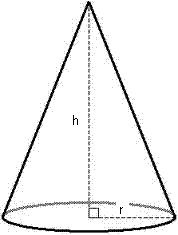# Cone Calculator

A geometrical shape that has a circular base and a single vertex called as Cone. In case vertex is over the center of the base is called a right cone otherwise it is called as oblique cone. Any object has cone shape is called conical.
Calculate the various properties of a cone for given values.Slant Height(l) of Cone: [ Sqrt(r² + h²) ] Enter the radius = Enter the height = Slant Height(l) of Cone =Volume of Cone: [ (1/3)πr²h ] Enter the radius = Enter the height = Volume of Cone =Curved Surface Area(CSA) of Cone: [ πrl ] Enter the radius = Enter the height = Curved Surface Area(CSA) of Cone =Total Surface Area(TSA) of Cone: [ πr(l+r) ] Enter the radius = Enter the height = Total Surface Area(TSA) of Cone =

>